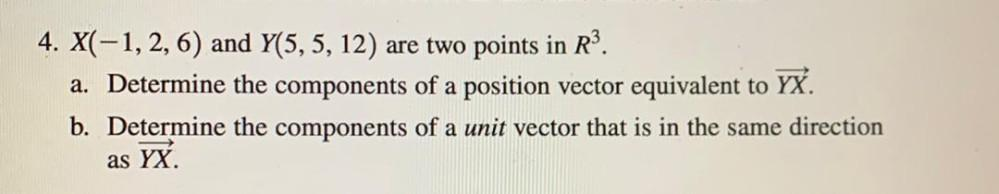Question:

# 4. X(-1, 2, 6) and Y(5, 5, 12) are two points in R³. a). Determine the components of a position vector equivalent to YX. b). Determine the components of a unit vector that is in the same direction as4. X(-1, 2, 6) and Y(5, 5, 12) are two points in R³. a). Determine the components of a position vector equivalent to YX. b). Determine the components of a unit vector that is in the same direction as YX.